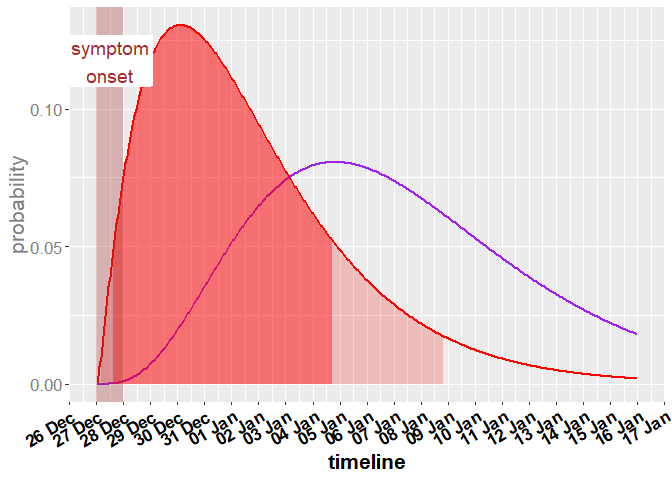# Statistical Modelling for Infectious Disease Management - Contacts

## Question

One person has become infected with COVID-19 on 28.12.2021. When will the contact person become ill and show the first symptoms?

## Generating a data frame with dates and illness probability of contacts using get_serial_interval_density

The function get_serial_interval_density() creates a dataframe containing the probability that a contact will start showing symptoms (serial interval) at a particular date/time. Therefore, only the symptom begin date of the infected person is needed. Furthermore, the probability when the infected contacts of infected contacts show symptoms can be calculated, known as the second and also third generation of contacts.

### Inputs

Multiple arguments are needed for the function get_serial_interval_density():

First of all, the symptom_begin_date has to be specified, which is defined as the date when the person started to show symptoms.

Furthermore, the max_serial_interval_days is needed, which defines the interval length of the distribution output.

The remaining two inputs shape_serial and rate_serial are the parameters of the log-normal distribution, which models the serial interval.

symptom_begin_date <- as.Date("2021-12-28")
max_serial_interval_days <- 20
shape_serial <- 2.154631545
rate_serial <- 0.377343528

serial_in_df_v1 <- get_serial_interval_density(symptom_begin_date,
max_serial_interval_days,
shape_serial,
rate_serial)

### Methodology

The default values of the parameters for the distribution, when the infected contacts will show symptoms, are from the paper Najafi et al. , in which a gamma distribution for the serial interval between symptom onsets of infected persons and the symptom onset of infected contacts was estimated.

For the second generation of contacts the probability equals the summation of random variables because we assume that the infection from infected person to the first contact generation is independent from the first to the second generation. Thus, a convolution of two identical gamma distributions has to be conducted. For gamma distributions the convolutions of gamma distributions equals the summation of their first parameters . Thus, the density for the second generation of infected persons showing symptoms is given by a gamma distribution with $$2 \cdot$$ shape_serial and the same rate_serial. In general, the serial interval for the $$i$$-th generation of contacts can be calculated with a gamma distribution with parameters $$i \cdot$$ shape_serial and rate_serial. This holds in an analogous way for the third generation.

### Output

The function call returns the following data set:

values 100 to 109 of resulting data frame
dates distribution
100 2022-01-01 04:00:00 0.1233038
101 2022-01-01 05:00:00 0.1227971
102 2022-01-01 06:00:00 0.1222783
103 2022-01-01 07:00:00 0.1217479
104 2022-01-01 08:00:00 0.1212064
105 2022-01-01 09:00:00 0.1206542
106 2022-01-01 10:00:00 0.1200916
107 2022-01-01 11:00:00 0.1195191
108 2022-01-01 12:00:00 0.1189372
109 2022-01-01 13:00:00 0.1183462

The data frame shows for each hour beginning at symptom_begin_date until max_serial_interval_days the resulting density of the gamma distribution. This density can be used for calculating the most probable period for a contact person start showing symptoms. The same data frame is obtained for the second generation. Here, the time interval of the distribution is larger.

symptom_begin_date <- as.Date("2021-12-28")
max_serial_interval_days <- 20
shape_serial <- 2 * 2.154631545
rate_serial <- 0.377343528

serial_in_df_v2 <- get_serial_interval_density(symptom_begin_date,
max_serial_interval_days,
shape_serial,
rate_serial)
values 100 to 109 of resulting data frame
dates distribution
100 2022-01-01 04:00:00 0.0383758
101 2022-01-01 05:00:00 0.0390547
102 2022-01-01 06:00:00 0.0397325
103 2022-01-01 07:00:00 0.0404089
104 2022-01-01 08:00:00 0.0410838
105 2022-01-01 09:00:00 0.0417569
106 2022-01-01 10:00:00 0.0424281
107 2022-01-01 11:00:00 0.0430971
108 2022-01-01 12:00:00 0.0437638
109 2022-01-01 13:00:00 0.0444279

## Visualization example of the data frame of get_serial_interval_density

The following code generates a plot with the gamma distribution of the illness probability of the first contact generation and the 80% and 95% high density intervals. In addition, the illness probability for the second generation is plotted in violet.

Source
.calculate_qstart_qend <- function(probability, df) {
hdr_df <- hdr(den = data.frame(x = 1:length(df$distribution), y = df$distribution),
p = probability * 100)$hdr qstart <- (hdr_df[1, 1] - 1) / 24 qend <- (hdr_df[1, 2] - 1) / 24 return(list("qstart" = qstart, "qend" = qend)) } Source .shade_curve <- function(df, qstart, qend, fill = "red", alpha = 0.4) { subset_df <- df[floor(qstart * 24):ceiling(qend * 24), ] geom_area(data = subset_df, aes(x = x, y = y), fill = fill, color = NA, alpha = alpha) } Source  symptom_begin_date <- as.Date("2021-12-28") df <- get_serial_interval_density(symptom_begin_date, max_serial_interval_days = 20, shape_serial = 2.154631545, rate_serial = 0.377343528) period_80 <- .calculate_qstart_qend(0.8, df) period_95 <- .calculate_qstart_qend(0.95, df) df_2 <- get_serial_interval_density(symptom_begin_date, max_serial_interval_days = 20, shape_serial = 2 * 2.154631545, rate_serial = 0.377343528) symp_date_posixct_start <- as.POSIXct(format(as.POSIXct(symptom_begin_date, tz = "CET"), "%Y-%m-%d")) symp_date_posixct_end <- as.POSIXct(format(as.POSIXct(symptom_begin_date + 1, tz = "CET"), "%Y-%m-%d")) symp_date_posixct_mid <- symp_date_posixct_start - as.numeric(difftime(symp_date_posixct_start, symp_date_posixct_end, units = "hours")) / 2 * 3600  Source  g <- ggplot() + scale_x_datetime(breaks = scales::date_breaks("1 days"), labels = scales::date_format("%d %b")) + theme(axis.text.x = element_text(angle = 90)) + # scale_x_continuous(breaks = x_tick, # labels = x_label) + # theme(axis.ticks.x = element_line(color = c(rbind(rep("black", length(x_label) / 2), rep(NA, length(x_label) / 2))), linetype = 2, size = 1)) + geom_path(aes(x = df$dates, y = df$distribution), color = "red", size = 1) + geom_path(aes(x = df_2$dates, y = df_2$distribution), color = "purple", size = 1) + .shade_curve(df = data.frame(x = df$dates, y = df$distribution), period_80$qstart,
period_80$qend) + .shade_curve(df = data.frame(x = df$dates, y = df$distribution), period_95$qstart,
period_95$qend, alpha = 0.2) + geom_rect(data = data.frame(xmin = symp_date_posixct_start, xmax = symp_date_posixct_end, ymin = -Inf, ymax = Inf), aes(xmin = xmin, xmax = xmax, ymin = ymin, ymax = ymax), fill = "brown", alpha = 0.3) + geom_label(aes(x = symp_date_posixct_mid, y = 0.9*max(df$distribution), label = "symptom\nonset"),
colour = "brown", fill = "white", size = 5, label.size = NA) +
ylab("probability") +
xlab("timeline") +
labs(color = 'Verteilung') +
# ggtitle("Visualization of get_infection_date_density ") +
theme(legend.position = "none", text = element_text(size = 16*5/5)) +
theme(axis.text.x = element_text(colour = "black", face = "bold", angle = 30, hjust = 1)) +
theme(axis.title.x = element_text(colour = "black", face = "bold")) +
theme(axis.text.y = element_text(colour = "gray50")) +
theme(axis.title.y = element_text(colour = "gray50"))

g## Literature

 Najafi F, Izadi N, Hashemi-Nazari S-S, Khosravi-Shadmani F, Nikbakht R, Shakiba E. Serial interval and time-varying reproduction number estimation for COVID-19 in western Iran. New Microbes and New Infections. 2020; 36: 100715.